# High School Chemistry : Help with Rate-Determining Steps

## Example Questions

### Example Question #7 : Kinetics

Consider the following reaction: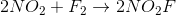The above reaction is actually composed of two steps that take place at different rates:

1.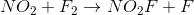(slow step)

2.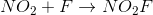(fast step)

Based on this, what is the rate law for the reaction?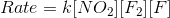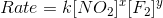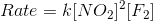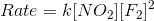Explanation:

For reactions with multiple steps, the step with the slowest rate will determine the rate law. In this reaction, the slowest step is the first step, so the reactants in that step will be the components of the rate law.

The first step is written as:Using the reactants, we can determine the rate law to beNote that we cannot determine the value of the exponents, since they will need to be found experimentally.

### Example Question #1 : Kinetics

The overall reaction can only proceed as quickly as the __________.

intermediate is decomposed

rate-determining step

products are removed